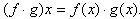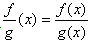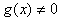Introduction

Multiplying and dividing functions will require more steps than adding and subtracting functions. While this topic will be covered more thoroughly in the Polynomial Unit, multiplying of linear terms and dividing by a monomial will be discussed here.

The function notation for multiplying and dividing is very similar to adding and subtracting. When multiplying functions,When dividing two functions,, wherebecause division by zero is an undefined operation in mathematics.

Listen